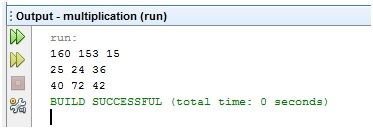# Java | Print Multiplication of two 2-D Arrays by traversing forward direction in first array and backward direction in second array

In this program, we are going to find multiplication of two matrices (two - d array) by traversing forward direction in first array and backward direction in second array using java program.
Submitted by Anamika Gupta, on June 18, 2018

Here, We have to find the multiplication of the two matrix by moving forward direction in row major (row by row) order in one matrix and by backward direction in row major order in second one i.e. multiplying first element of one matrix with the last element of second one, second element of first one with the second last element of second one and so on…

Example:

```First matrix (X)
8	    9	     5
5	    6	     3
4	    9	     7

Second matrix (Y)
6	     8	   10
12	     4	    5
3	    17	   20
```

We have to multiply like that,

First element of first matrix (X) with the last one of second matrix (Y) → 8*20 and save it to the first index of new matrix. Then , 9*17 and save it to the 2nd index of new one and so on...

The new Matrix Z is

```    160		153		15
24		25		36
40		72		42
```

Program:

```public class Multiplication
{
public static void main(String[] args)
{
//first matrix
int x[][]={{8,9,5},{5,6,3},{4,9,7}};
//second matrix
int y[][]={{6,8,10},{12,4,5},{3,17,20}};
//matrix which stores multiplication of the two
int z[][]=new int;

for(int i=0,j=2;i<3;i++,j--)
for(int k=0,l=2;k<3;k++,l--)
z[i][k]=x[i][k]*y[j][l];

for(int i=0;i<3;i++)
{
for(int j=0;j<3;j++)
System.out.print(z[i][j]+" ");
System.out.println();
}
}
}
```

Output